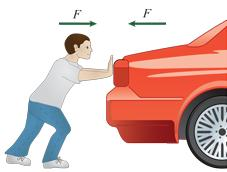# Conceptual Physics: IYou push a heavy car by hand. The car, in turn, pushes back on you. Doesn't this mean that the forces cancel each other, making acceleration impossible?

Why or why not?

A. Yes: In accord with Newton's second law, $a =\frac{F_\text{net}}{m}$, and since $F_{net}=F_{you}+F_{car}=0$, no acceleration occurs.

B. Yes: In accord with Newton's first and third laws, an object continues in its state of rest unless it is compelled to change that state by forces impressed upon it. Since the net force is zero, the car doesn't move.

C. No: In accord with Newton's third law, I push the car and the car pushes with an equal and opposite force on me. Thus, with Newton's second law and $a=\frac{F}{m}$, the car accelerates, but so do I in an equal and opposite direction.

D. No: An external, horizontal force is applied to the car by my push; the car accelerates (in accord with Newton's first law).


Note: By acceleration we mean acceleration relative to the ground.

Problem credit: Conceptual Physics: Special Edition Series, Paul G. Hewitt

×